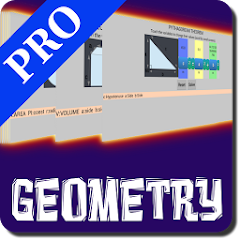# Interactive Geometry PRO

5+Everyone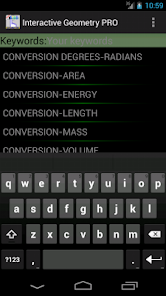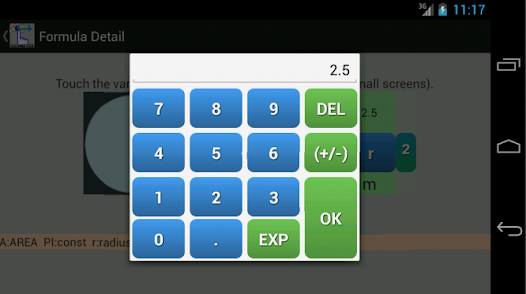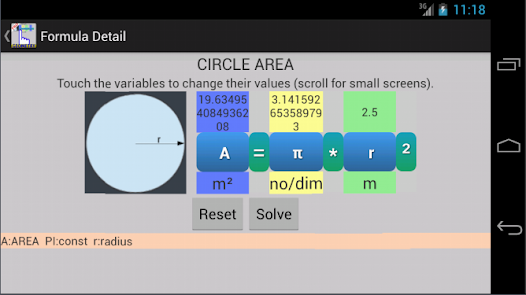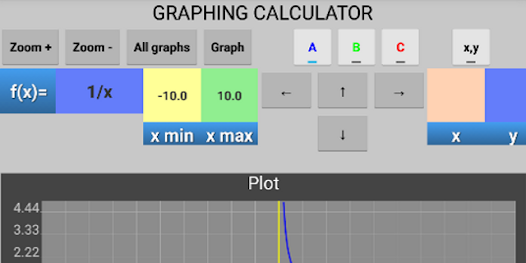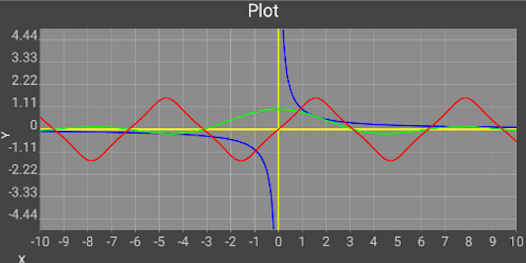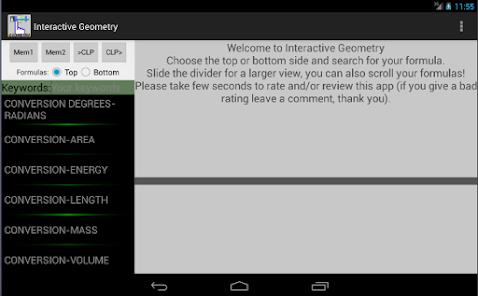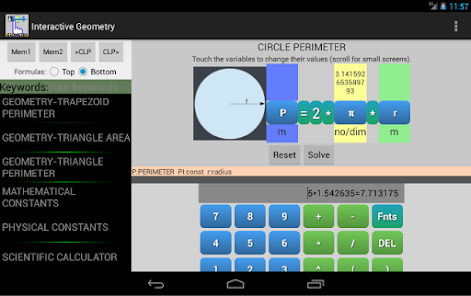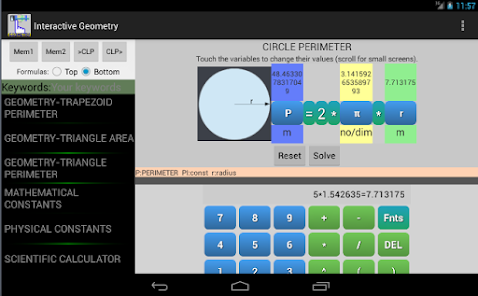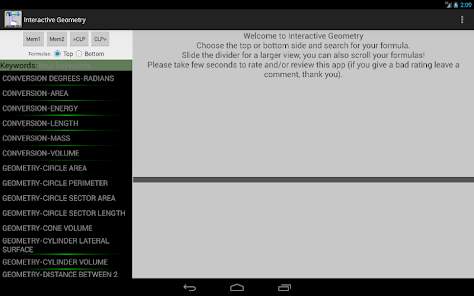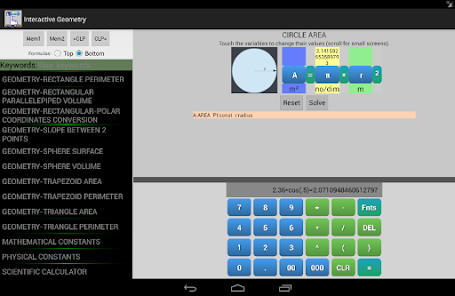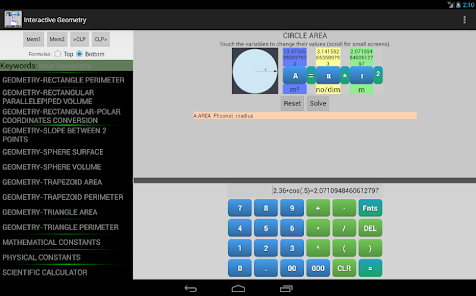"Interactive Geometry PRO" is ideal for students and graduates, you will find a variety of useful geometry formulas and you can enter a value by touch, the interactive feature make it easy to avoid typing or human errors.
The interface is made in a way that you can see above any variable it's value.
Topics:
"GEOMETRY-RECTANGLE PERIMETER",
"GEOMETRY-RECTANGLE AREA",
"GEOMETRY-TRIANGLE PERIMETER",
"GEOMETRY-TRIANGLE AREA",
"GEOMETRY-PARALLELOGRAM PERIMETER",
"GEOMETRY-PARALLELOGRAM AREA",
"GEOMETRY-TRAPEZOID PERIMETER",
"GEOMETRY-TRAPEZOID AREA",
"GEOMETRY-CIRCLE PERIMETER",
"GEOMETRY-CIRCLE AREA",
"GEOMETRY-CIRCLE SECTOR LENGTH",
"GEOMETRY-CIRCLE SECTOR AREA",
"GEOMETRY-ELLIPSE AREA",
"GEOMETRY-RECTANGULAR PARALLELEPIPED VOLUME",
"GEOMETRY-SPHERE SURFACE",
"GEOMETRY-SPHERE VOLUME",
"GEOMETRY-CYLINDER LATERAL SURFACE",
"GEOMETRY-CYLINDER VOLUME",
"GEOMETRY-CONE VOLUME",
"GEOMETRY-PYRAMID VOLUME",
"GEOMETRY-ELLIPSOID VOLUME",
"GEOMETRY-DISTANCE BETWEEN 2 POINTS",
"GEOMETRY-SLOPE BETWEEN 2 POINTS",
"GEOMETRY-RECTANGULAR-POLAR COORDINATES CONVERSION".
+ more than 110 other formulas.
Updated on
Nov 28, 2016

## Data safety